# XLOOKUP vs VLOOKUP in Excel - What's the Difference?Lydia Uys

## What are the XLOOKUP & VLOOKUP functions in Excel?

The XLOOKUP and VLOOKUP functions in Excel are used to find, or 'lookup', a value from a table or a list and then return a related result. In this resource, we’ll discuss XLOOKUP vs VLOOKUP with examples of how to use each.

Use this free Excel file to practice along with the tutorial.

In the image below you have a dataset on the left where members signed up for workshops, called the Workshop Registrations dataset. This dataset is missing some information about the members, for example, the “Last Name” field.

The information about the members can be found in another dataset, called the Members dataset, and can be retrieved from there to complete the required fields in the Workshop Registrations dataset.

To get the information from one dataset to complete the other, you would normally use a VLOOKUP. If you have access to Office 365, you could consider the new XLOOKUP to get the job done instead.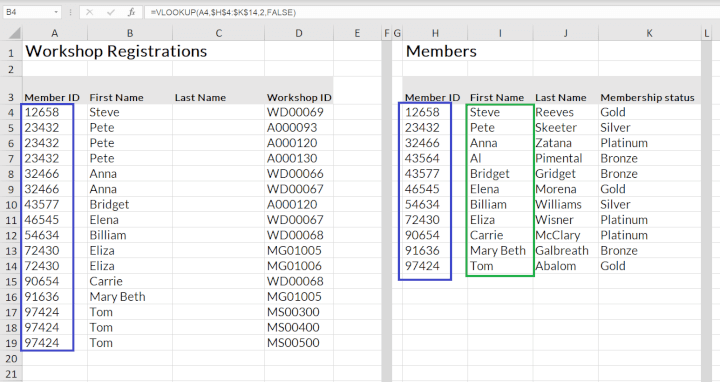How you look up the member’s detail in the dataset is a little different for XLOOKUP and VLOOKUP. Let's take a look at the syntax and differences of each.

## Syntax

Both formulae require a minimum of three arguments. The square brackets indicate the optional arguments.

=vlookup(lookup_value, table_array, col_index_num, [range_lookup])

=xlookup(lookup_value, lookup_array, return_array, [if_not_found], [match_mode], [search_mode])

### XLOOKUP vs VLOOKUP: Comparison table

VLOOKUP XLOOKUP
Exact match default N Y
Return value to the right of lookup Y Y
Return value to the left of lookup N Y
Lookup in row N Y
Return more than one value N Y
Lookup column needs to be sorted Approximate match only Binary search only
Search from top to bottom Y Y
Search from bottom to top N Y
Binary search N Y
Wildcard search Y Y
Exact search Y Y
Approximate search returning next smaller value Y Y
Approximate search returning next larger value N Y
May return an incorrect value if lookup values are not sorted? Y - approximate

N - approximate,

Y - Binary

Add new columns to search table may break the formula Y N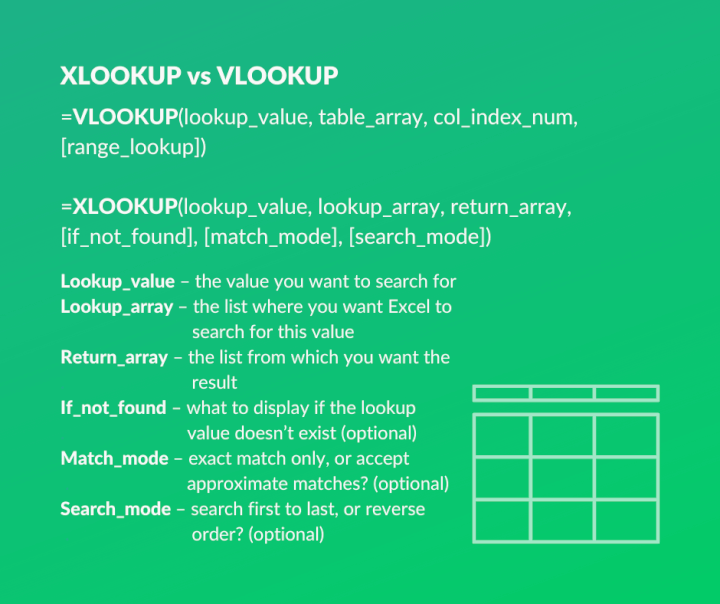### Lookup value

The value you want to find on the new list (e.g. the member ID). Both VLOOKUP and XLOOKUP usually lookup (search for) a single value.

### Table array (VLOOKUP) / Lookup array (XLOOKUP)

This represents the biggest difference between VLOOKUP and XLOOKUP and in the example above will be the member’s dataset where you would find both the member (lookup value) and the member’s first name or last name (returned value).

The VLOOKUP uses table_array, this area indicates the column for the lookup value and stretches to include the column for the returned value. The next argument (return array) refers back to this array by indicating the relevant column number of the table array that houses the return value.

Excel is programmed to search for the lookup value in the left column of this range and return a value to the right of this, indicated by the column number from the next argument.

The XLOOKUP separates the lookup array and the return array into two arguments. The lookup array should house the value Excel will search for and the return array argument will house the value to be returned.

The separation of the arrays makes the XLOOKUP much more flexible than the VLOOKUP. The lookup array can now be to the left or the right of the return value column and the XLOOKUP can act as a VLOOKUP or an HLOOKUP.

### Column index number (VLOOKUP) / Return array (XLOOKUP)

This argument indicates the range where the returned value can be found. In our example, this would be the first name of the member.

In a VLOOKUP this is a single number that refers back to the table array (the previous argument that indicated the entire range where the search value and returned value can be found).

The VLOOKUP is easy to ‘break’ if this column number is hardcoded into the argument, which is an important benefit to the XLOOKUP.

The XLOOKUP requires an entire range of values for this argument to indicate the array where the return value may be found. This range has to be aligned with the lookup array (previous argument) in length and position to avoid a #VALUE! error.

The return array in the XLOOKUP can also accommodate more than one return column in the return array, which will return more than one value related to the lookup value. You will have to use two VLOOKUPs to manage the same.

The past two arguments (the separation of the ranges) indicate the biggest benefits to the XLOOKUP:

• The lookup array can be to the right or left of the return array
• The XLOOKUP is harder to break because the two (lookup and return) arrays are relative and not hardcoded into the formula.

This is where users rave about the XLOOKUP and are ready to dispose of the VLOOKUP immediately.  BUT, if you don’t hard code the column number in the VLOOKUP, but manage to make this argument the result of a formula, the VLOOKUP will provide a benefit that may be hard to replicate with an XLOOKUP alone.

## Optional arguments

If the XLOOKUP cannot find a value, it returns a default or a customized message. The message may read, “The member you are looking for is not registered in our database.”

Unless you add an IFERROR, the VLOOKUP will return its usual #N/A! (not available) error, which may make you believe you did something wrong and the VLOOKUP is not working.

### Range lookup (VLOOKUP) / Match mode (XLOOKUP)

Exact (false/0) and approximate (true/1) matches in VLOOKUP have similar possibilities in XLOOKUP. Compared, it would look like this:

XLOOKUP programmed options

VLOOKUP options that perform similarly

Exact (0) - Default Exact (1)
Approximate (exact or next smaller value) (-1) Approximate – Default
Approximate (exact or next larger value) (1) None
Wildcard (?*~) (2) Exact (1) match using the same wildcard characters

The XLOOKUP defaults to an exact match where the VLOOKUP defaults to an approximate match. As the exact match is used most often, this setting would make the XLOOKUP more effective. On top of this, the XLOOKUP offers an additional option of an approximate match returning the next larger value.

### Search mode (XLOOKUP)

The VLOOKUP is programmed to search from top to bottom (first to last) and will return the first matching item for exact and approximate matches.

The XLOOKUP has four possible search options:

• First to last (1) - default
• Last to first (-1)
• Binary search - assume ascending order sort for lookup range (2)
• Binary search - assume descending order sort for lookup range (-2)

The methods of searching open up additional functionality for the XLOOKUP - you can search for the last occurrence of an item in a range. The binary search method is faster in sheets with large quantities of data but relies on the fact that the data in the lookup range is sorted.

The VLOOKUP searches from first to last and can only look from last to first with the use of some serious maneuvering in Excel.

## Other differences

### Sorting of lookup range

The VLOOKUP approximate (true) search will return incorrect values if the lookup range is not sorted in ascending order.

The XLOOKUP is inhibited by sorting when it searches from last to first or when it uses binary search.

### Compatibility with older versions of Excel

VLOOKUP has no compatibility issues with older versions of Excel. XLOOKUP is only available in Office 365, roll out to other versions planned to start in July 2020.

If you want to share a worksheet with XLOOKUPs with collaborators, make sure they also have access to Office 365 for the time being.

### Horizontal lookup

The XLOOKUP not only improves the VLOOKUP functionality but also replaces the HLOOKUP.

## Troubleshooting

### Inclusion of headings in the lookup range

It is always risky to include headings from the lookup range in the VLOOKUP or the XLOOKUP as Excel may interpret the heading as part of the data and may return incorrect values that seem correct as a result.

### Error messages

#### VLOOKUP:

#N/A - value searched for is not available.

• Example: the member number cannot be found in the member dataset.

#REF - the value to return is outside of the defined table array.

• Example: you selected five columns and want a return value from column 6.

#### XLOOKUP:

#N/A - value searched for is not available.

• Example: the member number cannot be found in the member dataset. (Same as VLOOKUP.)

#REF - the other workbook as referred to in the formula is not open.

#VALUE - the dimensions of the lookup array and return array are not aligned in position and length.

• Example: the lookup array is five rows long and the return array is four rows long, or the lookup array is in a row and the return array is in a column.

## When to use XLOOKUP or VLOOKUP

XLOOKUP can be used for the same tasks as VLOOKUP and then has a few extra uses. It may be better to show you examples.

### Example 1 - Find a value that exists and is unique

The first example looks for a member number that exists (exact match) in the members dataset, and is unique - a value that you would expect to find easily.

B4: =VLOOKUP(A4,\$J\$4:\$L\$14,2,FALSE)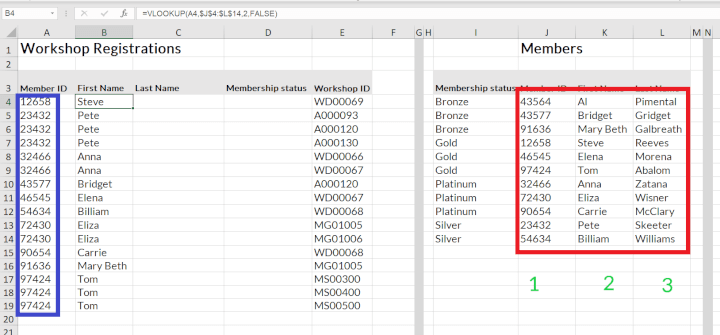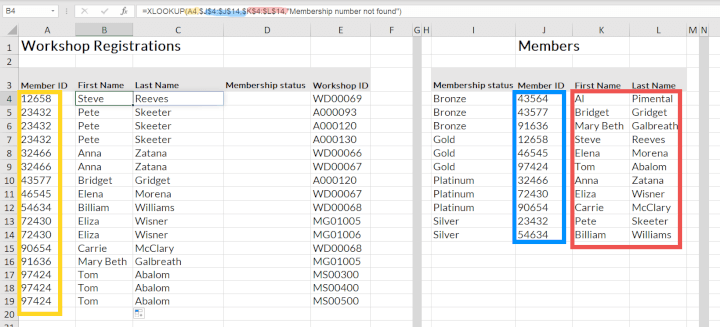Note: The lookup values are the same. The range for the VLOOKUP includes the entire column, but the XLOOKUP splits the referenced ranges to a range to search and one to find the returned value.

Also note that the XLOOKUP used one formula to return two values.

### Example 2  - Find a value that does not exist

Excel is required to find a member that does not exist in the member’s dataset to produce the first name.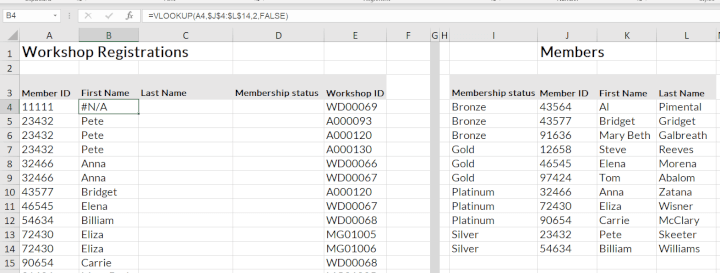#### VLOOKUP

The exact VLOOKUP returns a #N/A - which translates to not available.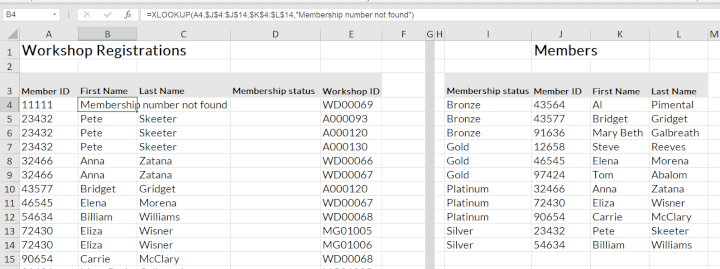#### XLOOKUP

XLOOKUP exact match only finds nothing, as expected, and returns a customized message.

### Example 3 - Find a value to the left

In this example, Excel has to look for the value 4 in the column named ‘99’ and return the associated ‘number’ to the left.

VLOOKUP cannot comply, so an alternative index and match can be used.

The XLOOKUP is fairly simple in comparison.

D4: =XLOOKUP(A4,\$J\$4:\$J\$14,\$I\$4:\$I\$14)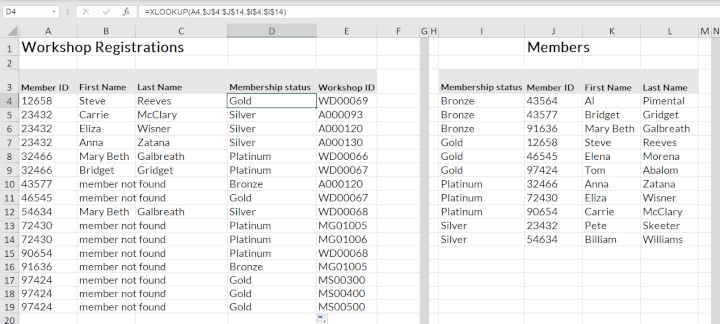### Example 4 - Find the last available occurrence of a value

This example shows a list of customer orders. If we wanted to know what the last order total was for a certain customer, we could use the new XLOOKUP last to first search functionality. In this example the database needs to be sorted by date.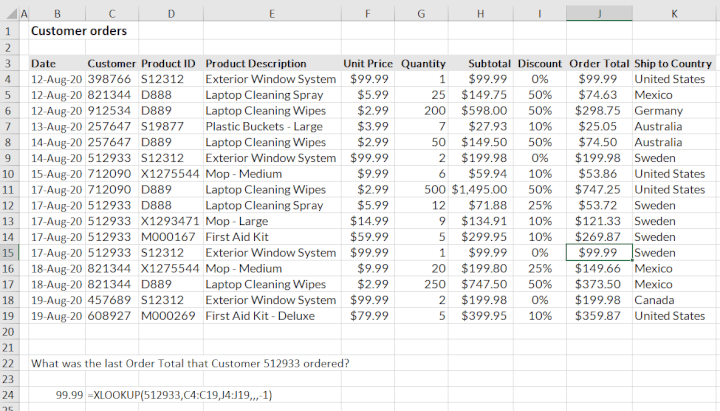## Conclusion

So there you have it, a breakdown of XLOOKUP vs VLOOKUP.

The XLOOKUP is a definite improvement to the VLOOKUP and replaces a lot of the bypasses that you had to rely on to get a VLOOKUP to work in certain instances (INDEX, MATCH, IFERROR, and other maneuverings).

The XLOOKUP is unfortunately not available for older versions of Excel, and you will have to keep this in mind when sharing worksheets.

VLOOKUP was a trusted old companion and it may still have a place in certain instances - so don’t write it off yet. But the XLOOKUP is without a doubt a great and much-needed improvement.

To learn more about XLOOKUP, VLOOKUP, and other essential functions in Excel, try our Excel - Basic and Advanced course now. Or start with the free Excel in an Hour course to learn some basics in Excel.

Free Excel crash course

Learn Excel essentials fast with this FREE course. Get your certificate today!Lydia Uys

Lydia is a Chartered Accountant and has experience in financial management, lecturing, and consulting. She currently creates content for GoSkills and also loves spending time with family and friends.

1 comment

•Tavia

Great functions, can't decide which is better!